• 定义兼容性对象数组T 表示传入的类型，可以为number、string、boolean等传入什么label就是什么类型interface labelValue {label: T;value: number;}// 传入number类型let obj: labelValue;export function ...
定义兼容性对象数组T 表示传入的类型，可以为number、string、boolean等传入什么label就是什么类型interface labelValue {label: T;value: number;}// 传入number类型let obj: labelValue;export function formatYear (): labelValue[] {let date = new Date().getFullYear();let arr: labelValue[];for (let i = 0; i < date + 10; i++) {obj = {label: i,value: i}arr.push(obj)}return arr;}// 传入string类型let obj1: labelValue;export function formatHours (): labelValue[] {let arr: labelValue[];for (let i = 0; i <= 24 + 10; i++) {obj1 = {label: i + 'h',value: i}arr.push(obj1)}return arr;}定义对象型数组定义interface ObjectOf{[_: string]: V}class OrderItem {uuid: string;amount: number;secondaryTotal: number;total: number;originalTotal: number;checked: boolean;}使用interface State {promoList: {giftCheck: ObjectOffreeCheck: Array}}
展开全文• 对象类型基础使用在 TypeScript 中，我们使用接口(Interfaces)来定义对象的类型。简单例子：interface Person {name: string;age: number;}let tom: Person = {name: 'Tom',age: 25};复制代码上面的例子中，我们定义...
对象类型基础使用在 TypeScript 中，我们使用接口(Interfaces)来定义对象的类型。简单例子：interface Person {name: string;age: number;}let tom: Person = {name: 'Tom',age: 25};复制代码上面的例子中，我们定义了一个接口 Person，接着定义了一个变量 tom，它的类型是 Person。这样，我们就约束了 tom 的形状必须和接口 Person 一致。定义的变量比接口少/多了属性是不允许的：interface Person {name: string;age: number;}let tom: Person = {name: 'Tom'};let tom: Person = {name: 'Tom',age: 25,gender: 'male'};//都会报错哦复制代码可选属性但是有些时候，我们并不希望对象的形状完全遵守接口的定义(我们希望少/多些属性)：所以我们会用到可选属性interface Person {name: string;age?: number;}let tom: Person = {name: 'Tom'};// 特征是打了个问号，意味着并不是必须的，而是可选的属性//但是这个时候可以少属性，却不能多属性复制代码任意属性我们会使用任意属性来实现可以少属性/多属性interface Person {name: string;age?: number;[propName: string]: any;}let tom: Person = {name: 'Tom',gender: 'male'};复制代码需要注意的是，一旦定义了任意属性，那么确定属性和可选属性的类型都必须是它的类型的子集:interface Person {name: string;age?: number;[propName: string]: string; // 任意属性只包含了string类型}let tom: Person = {name: 'Tom',age: 25, // age的nubmer属性不在任意属性string内，会报错gender: 'male'};复制代码只读属性有时候我们希望对象中的一些字段只能在创建的时候被赋值，那么可以用 readonly 定义只读属性：interface Person {readonly id: number;name: string;age?: number;[propName: string]: any;}let tom: Person = {id: 89757,name: 'Tom',gender: 'male'};tom.id = 9527;// 该属性只读，赋值操作会报错复制代码数组类型数组类型会对其的内容进行约束「类型 + 方括号」表示法let fibonacci: number[] = [1, 1, 2, 3, 5];复制代码数组泛型let fibonacci: Array = [1, 1, 2, 3, 5];复制代码用接口(interface)表示数组interface NumberArray {[index: number]: number;}let fibonacci: NumberArray = [1, 1, 2, 3, 5];复制代码类数组不能使用以上3钟形式去表示function sum() {let args: {[index: number]: number;length: number;callee: Function;} = arguments;}复制代码函数类型函数声明函数表达式在 JavaScript 中，有两种常见的定义函数的方式如上。一个函数有输入和输出，所以基本上就是把约束覆盖到函数的输入与输出上。1. 传统的定义函数的输入与输出// 函数声明的写法function sum(x: number, y: number): number {return x + y;}// 多参数 | 少参数 是不被允许的哦// 函数表达式的写法，等式左边的可以被省略而完全又右边类型判断出来。let mySum: (x: number, y: number) => number = function (x: number, y: number): number {return x + y;};复制代码2. 用接口定义函数的输入与输出interface SearchFunc {(source: string, subString: string): boolean;}let mySearch: SearchFunc = function(source: string, subString: string) {return source.search(subString) !== -1;}复制代码- 函数的参数详情前面我们提到，输入多/少的参数式不被允许的，这在接口定义中也存在着这个限制，同样的，我们也可以使用？来定义可选参数！！！可选参数必须接在必需参数后面，否则会报错。如下是正确写法function buildName(firstName: string, lastName?: string) {if (lastName) {return firstName + ' ' + lastName;} else {return firstName;}}let tomcat = buildName('Tom', 'Cat');let tom = buildName('Tom');复制代码参数也可以拥有默认值, TypeScript 会将添加了默认值的参数识别为可选参数,此时就不受「可选参数必须接在必需参数后面」的限制了：function buildName(firstName: string, lastName: string = 'Cat') {return firstName + ' ' + lastName;}let tomcat = buildName('Tom', 'Cat');let tom = buildName('Tom');复制代码-  函数的重载这个挺……无语的，感觉很没必要function reverse(x: number | string): number | string {if (typeof x === 'number') {return Number(x.toString().split('').reverse().join(''));} else if (typeof x === 'string') {return x.split('').reverse().join('');}}复制代码本教程完全依赖于 ： https://ts.xcatliu.com/introduction/index.html复制代码
展开全文• TypeScript 数组类型最简单的方法...数组的项中不允许出现其他的类型数组的一些方法的参数也会根据数组定义时约定的类型进行限制：let fibonacci: number[] = [1, 1, 2, 3, 5];fibonacci.push('8');// index.t...
TypeScript 数组的类型最简单的方法是使用「类型 + 方括号」来表示数组let fibonacci: number[] = [1, 1, 2, 3, 5];数组的项中不允许出现其他的类型：数组的一些方法的参数也会根据数组在定义时约定的类型进行限制：let fibonacci: number[] = [1, 1, 2, 3, 5];fibonacci.push('8');// index.ts(2,16): error TS2345: Argument of type 'string' is not assignable to parameter of type 'number'.上例中，push 方法只允许传入 number 类型的参数，但是却传了一个 string 类型的参数，所以报错了。数组泛型定义let fibonacci: Array = [1, 1, 2, 3, 5];用接口表示数组interface NumberArray {[index: number]: string;}let fibonacci: NumberArray = ["a","n"];NumberArray 表示：只要 index 的类型是 number，那么值的类型必须是 stringany在数组中的应用let list: any[] = ['Xcat Liu', 25, { website: 'http://xcatliu.com' }];类数组类数组(Array-like Object)不是数组类型，比如 arguments：function sum() {let args: number[] = arguments;}// index.ts(2,7): error TS2322: Type 'IArguments' is not assignable to type 'number[]'.//   Property 'push' is missing in type 'IArguments'.事实上常见的类数组都有自己的接口定义，如 IArguments, NodeList, HTMLCollection 等function sum() {let args: IArguments = arguments;}
展开全文• 基本类型类型注解作用：相当于强类型语言的类型声明... let str:string = 'asd'如果将str变量改成number类型，就会提示报错，ts不允许改变变量的数据类型数组类型两种定义方式 //数组类型 let arr1:number[] = [1...基本类型类型注解作用：相当于强类型语言的类型声明语法：(变量/函数)：type原始类型 //原始类型 let bool:boolean = true; let num:number = 123; let str:string = 'asd'如果将str变量改成number类型，就会提示报错，ts不允许改变变量的数据类型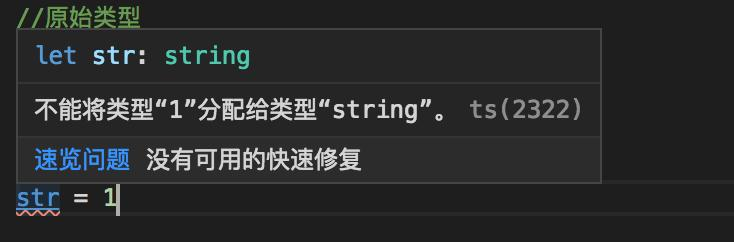数组类型两种定义方式 //数组类型 let arr1:number[] = [1,2,3] let arr2:Array = [1,2,3]ts只允许数组中包括一种数据类型的值，在数组arr1中添加字符串类型的值就会报错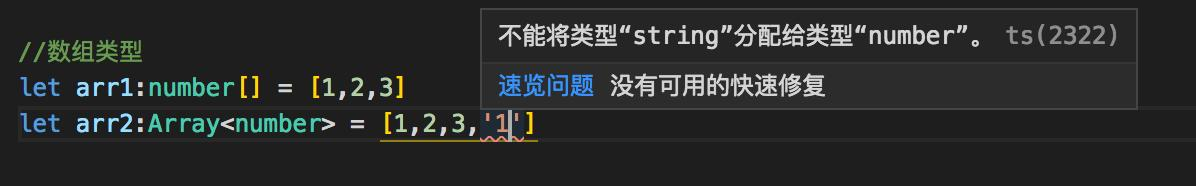如果想为数组添加不同类型的值，需要使用联合类型 //联合类型 let arr1:number[] | string[] = [1,2,3] let arr2:Array = [1,2,3]元组类型限定了数组的类型和个数，数组值必须安装声明变量时的类型一致，个数必须一致，否则都会报错。 let tuple:[number,string] = [1,'a'] //正确 let tuple2:[number,string] = ['a','a'] //错误，不能将字符串a赋值给nuumber let tuple3 = [number,string] = [1,'a',3] //错误，数组中只能有两个值元组越界问题:向元组中push一个值 let tuple:[number,string] = [1,'a'] tuple.push(2) console.log(tuple) //[1,'a',2],可以看出可以向tuple使用push方法添加  //但是不能访问，会报错。 tuple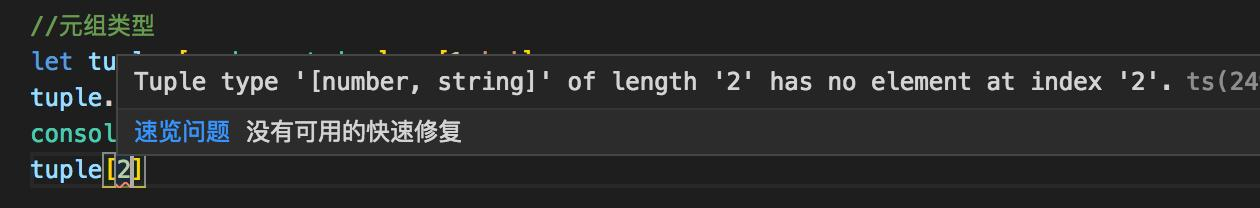函数 let add = (x:number,y:number):number => x+y;ts要求给函数参数提供类型值，返回值一般不需要提供类型值，ts会自动使用推导出返回值类型。对象 let obj:object = { a:1, b:2, }  // 这样写不允许修改对象的变量值 obj.a = 3 //报错  正确做法：给变量直接赋值对应的类型 let obj2:{a:number,b:number} = { a:1, b:2, } obj2.a = 3 //正确Symbol具有唯一的值两种定义方式 let s1:symbol = Symbol() let s2 = Symbol()  //此时s1和s2是不相等的 console.log(s1 === s2) //falsenull 和 undefined let nu:null = null; let un:undefined = undefined;void让任何表达式返回undefined没有任何返回值 let noReturn = () => {}any可以是任何类型的值never永远不会有返回值 let error = () => { throw new Error('err') }  let endless = () => { while(true){  } }枚举类型一组有名字的常量集合数字枚举使用enum定义枚举 // 正常枚举值是从0开始 enum Role { Reporter, //0 Developer, //1 Main, //2 Owner, //3 Guest, //4 } console.log(Role.Reporter) //0  //自定义枚举值，自定义后的值，需要一次往下递增 enum Role2 { Reporter == 1, //1 Developer, //2 Main, //3 Owner, //4 Guest, //5 }打印一下Role，是个对象。可以根据key和value索引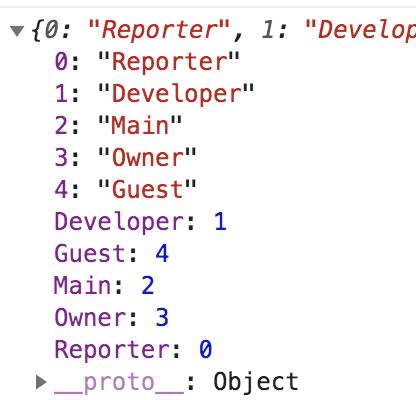枚举的实现原理：反向映射,看下图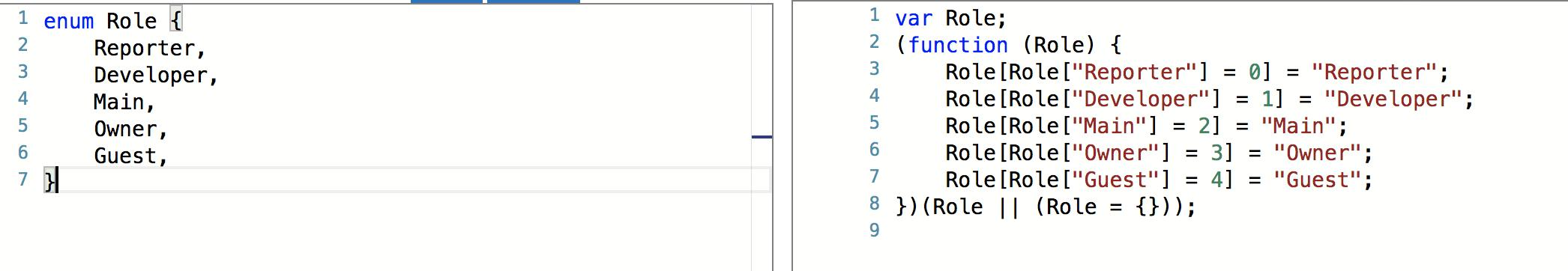字符串枚举 enum Msg { Success = '成功', Erros = '失败' }字符串枚举不可以反向映射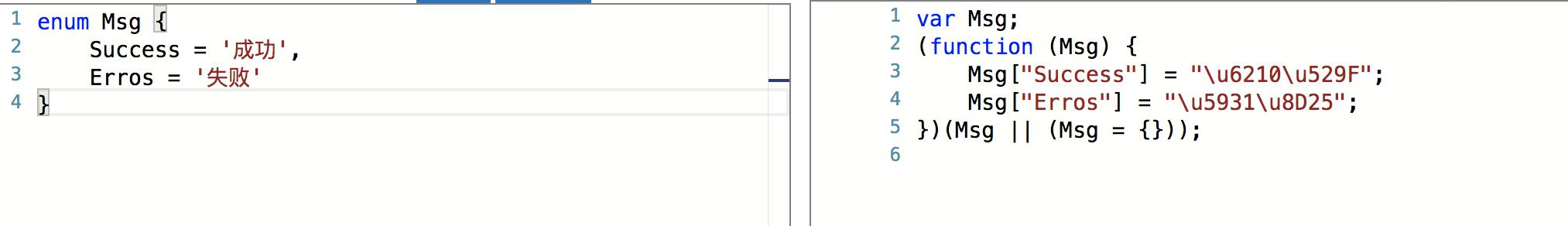异构枚举就是字符串枚举和数字枚举混用,不推荐使用 enum Answer { N, Y = 'yes' }枚举成员性质不能修改定义后的枚举值 enum Char { //常量枚举 a, b = Char.a, c = 1+ 3, //需要被计算的枚举成员 d = Math.random(), e = '123'.length }常量枚举(三种):会在编译时编译出结果没有初始值对已有枚举成员的引用常量表达式需要被计算的枚举成员在执行时才会计算出结果看下编译结果常量枚举：在编译时编需要被计算的枚举成员：没有编译出结果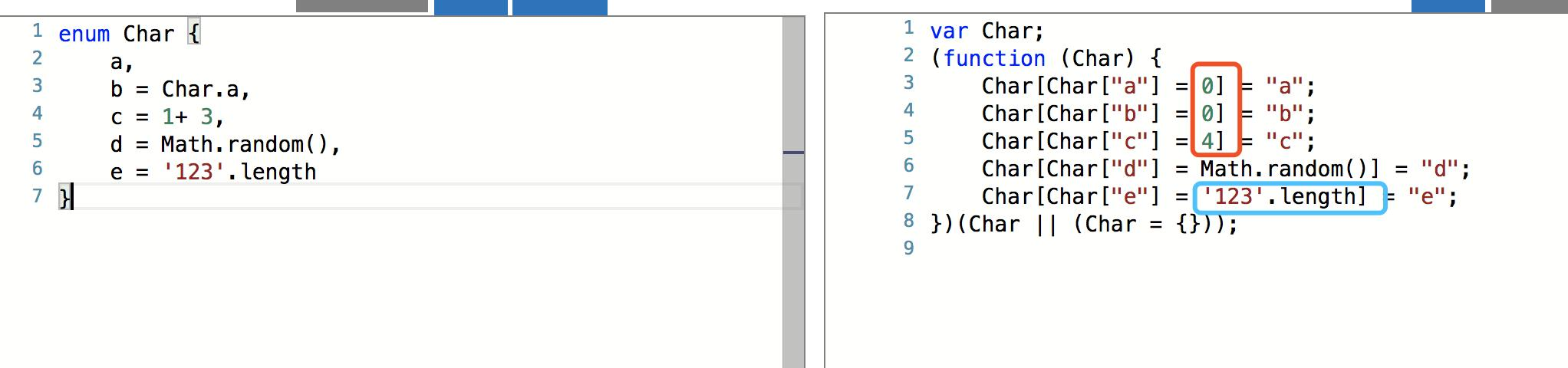常量枚举用const声明的枚举 const enum Month { Jan, Feb }会在编译阶段会被移除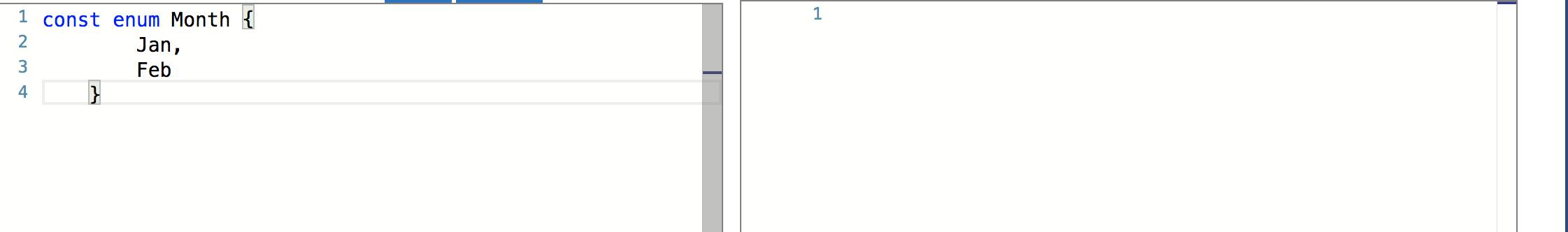枚举类型 enum E {a,b} //没有值 enum F {A=0,B=1} //数字枚举 enum G {a='a',b='b'} //字符串枚举
展开全文• 少侠们，今天我们继续来搞一搞TS今天我们要来看一看TS中数组的定义是个什么鬼样子数组的类型：在 TypeScript 中，数组类型有多种定义方式，比较灵活。下面我们来看看有哪些定义方法「类型 + 方括号」表示法：最简单...
• 展开全部数组定义：1、类型 + 方括号：比如：　636f70793231313335323631343130323136353331333431373933let tsArray: number[] = [1,1,2,3,4]2、使用数组泛型Array来表示数组：比如：let fibonacci: Array = [1, ...
• TS中的常用的数据类型有 (内置类型)Number, String, Boolean, Null, Undefined， Void, enum，Array，Any，Object，元组， 接口等数字类型例如 let decimal: number = 6;字符串let color: string = "blue";布尔类型...
• 第一步tsc--inti生成tsconfig.json改"outDir":"./js",2、第二步任务-运行任务监视tsconfig.json2、typeScript中的数据类型typescript中为了使编写的代码更规范，更有利于维护，增加了类型校验，在typescript中主要给...
• **1、空值**JavaScript 没有空值(Void)的概念，在 TypeScript 中，可以用 void 表示没有任何返回值的函数：...}*******2、Typescript基础操作**`//使用 string 定义字符串类型：let myName: string = 'T...
• typescript基础数据类型布尔值示例代码最基本的数据类型就是简单的true/false值，在JavaScript和TypeScript里叫做boolean(其它语言中也一样)。let a: boolean = trueconsole.log(a)let b: boolean = falseconsole....
• 先看一个TS都有什么类型let str: string = '字符串类型' // 字符串类型 ...Array 数组类型（只能是单一类型，例如string或者number）let arr: numer[] = [11, 22, 33] // number表示数字类型 后...
• 这些浮点数的类型是 number。 除了支持十进制和十六进制字面量，TypeScript 还支持 ECMAScript 2015 中引入的二进制和八进制字面量。let decLiteral: number = 6;let hexLiteral: number = 0xf00d;let ...
• 二、基础类型布尔类型let isDone: boolean = false;数字类型 数字类型包括：十进制，十六进制，二进制，八进制let decLiteral: number = 6; let hexLiteral: number = 0xf00d; let binaryLiteral: number = 0b1010; ...
• TypeScript是JavaScript的加强版，它给JavaScript添加了可选的静态类型和基于类的面向对象编程，它拓展了JavaScript的语法。而且TypeScript不存在跟浏览器不兼容的问题，因为在编译时，它产生的都是JavaScript代码。...
• 2019-10-28:学习内容：接口、数组类型、函数的类型(展开涉及多个内容)一、接口(Interfaces)：(1)LabelledValue接口就好比一个名字，用来描述上面例子里的要求。 它代表了有一个label属性且类型为string的对象。 ...
• 本文将通过一个简单的例子介绍如何为 TSLint 编写自定义规则，在开始之前默认读者对 Typescript 有所了解。本文例子源码前言它是什么？与 ESLint 一样属于程序静态分析工具(linter)，不过因为 Typescript 与 ...
• 注：本篇适合初学TS的宝宝们，众所周知，TS的写法对于数据类型要求比较严格，同时可维护性比较好， 所以很多大厂提倡用TS书写代码，那么，初学代码的时候几乎都是从数据类型方面入手，长话短说，下面 是一张ES6中常...
• PS: 本文语境下的「约束」指的是「类型对值的约束」一、构造类型抽象在 TS 的世界里，总有「动态地生成类型」的需求，比如下面的 UserWithHisBlogs 和 User 重复的部分:type User = {id: number;name: string...
• 很重要这一节很重要, 可以说是ts的最核心部分, 这一节学完其实就可以开始用ts写代码了, 想想typescript中的type, 再看看标题中的"类型"2字, 所以请大家务必认真.什么是入门高级类型因为高级类型的内容比较多, 但是...
• Vue3和TS的概念学了那么多，有没有心动手痒想实践一下呢？本次千字长文将手把手介绍如何使用Vue3+TS写一个基础项目，有了主要框架概念之后，后续对于应用的开发能更加地得心应手。1.版本升级大家之前在做Vue开发时...
• 定义类型： interface ObjectOf<V> { [_: string]: V } class OrderItem { uuid: string; amount: number; secondaryTotal: number; total: number; originalTotal: number; checked: boolean; } 使用...typescript
• ts 数组解构时定义类型Today I will talk about destructuring an array, first introduced in the ES6 version of Javascript. Destructuring is a way of extracting values from an array into variables. 今天，...
• 在 TypeScript 中，数组类型有多种定义方式，比较灵活。下面我们来看看有哪些定义方法 　「类型 + 方括号」表示法： 　最简单的方法是使用「类型 + 方括号」来表示数组：　 let tsArray: number[] = [1,1,2,3,4...
• 首先出现的问题是，我需要一个数组list，数组中每一项需要是key：value形式的...这里就需要使用TS的接口，我们需要自定义一下list数组类型，使用接口就可以自定义list中属性的类型。 先定义接口： interface Iitem...vue
• // 布尔类型 (Boolean) let flag: Boolean = true //数字类型 (Number) ...// 数组类型 (Array) // 1 let arr: number[] = [1, 2, 3, 4, 5] // 2 let arr1: Array<String> = ["6", "7", "8", "9",
• ## ts数据类型的定义

千次阅读 2019-11-16 21:00:26
1. 数据类型 let title = '张三' let age = 18 let loading = true 对比的写法 let title:string = '张三' let age:number = 18 ...定义数组 let arr = [1, 2, 3] 对比的写法 let arr:number = [1, 2, 3] let a...typescript